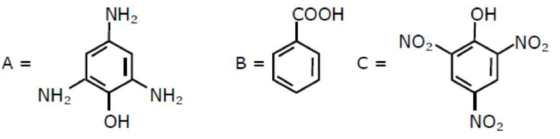# Compound(s) which will liberate carbon dioxide with sodium bicarbonate solution is/are:

Question:

Compound(s) which will liberate carbon dioxide with sodium bicarbonate solution is/are:1.  $\mathrm{B}$ and $\mathrm{C}$ only

2. B only

3.  $A$ and $B$ only

4. $C$ only

Correct Option:

Solution:

The number of orbitals with $\mathrm{n}=5, \mathrm{~m}_{1}=+2$ is_____________

(Round off to the Nearest Integer).